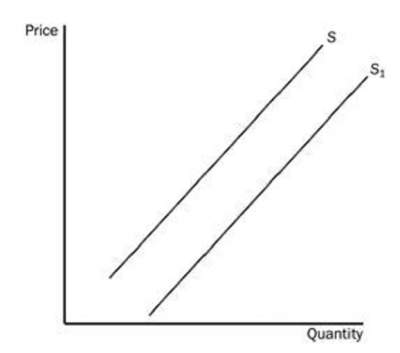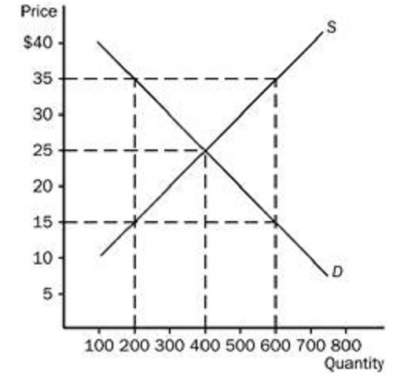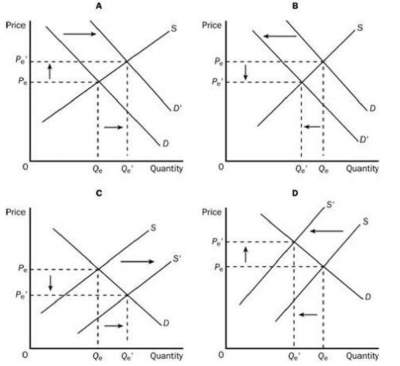# Practice Test-2 on Elasticity and its Application

Question 1: The mainstream view among economists is that .......................
1. no tradeoff exists between unemployment and inflation, either in the short run or in the long run.
2. society faces a tradeoff between unemployment and inflation, but only in the short run.
3. society faces a tradeoff between unemployment and inflation, but only in the long run.
4. society faces a tradeoff between unemployment and inflation, both in the short run and in the long run.
Question 2: The negative relationship between price and quantity demanded .....................
1. is represented by a downward-sloping demand curve.
2. applies to most goods in the economy.
3. is referred to as the law of demand.
4. All of the above are correct.
Question 3: Which of the following would not be a determinant of the demand for a particular good?
1. taxes
2. income
3. tastes
4. prices of related goods
5. the prices of the inputs used to produce the good
Question 4: If a good is normal, then an increase in income will result in ............................
1. a decrease in the demand for the good.
2. an increase in the demand for the good.
3. a movement up and to the left along the demand curve for the good.
4. a movement down and to the right along the demand curve for the good.
Question 5: Two goods are substitutes if a decrease in the price of one good ..............................
1. decreases the quantity demanded of the other good.
2. increases the demand for the other good
3. decreases the demand for the other good.
4. increases the quantity demanded of the other good.
Question 5: Two goods are complements if a decrease in the price of one good .........................
1. increases the demand for the other good.
2. decreases the quantity demanded of the other good.
3. decreases the demand for the other good.
4. increases the quantity demanded of the other good.
Question 6 : The movement from S to S1 in figure below is called1. an increase in supply.
2. a decrease in supply.
3. a decrease in quantity supplied.
4. an increase in quantity supplied
Question 7: At what price would there be an excess demand amounting to 200 units of the good?1. \$17
2. \$21
3. \$19
4. \$20
Question 8: Which of the four graphs represents the market for coffee after a major hurricane hits the coffee-growing area of the world?1. B
2. D
3. B
4. C
Question 9: Which of the four graphs (see graphs in question 8) illustrates an increase in quantity supplied?
1. B.
2. C.
3. D.
4. A.
Question 10: The price elasticity of demand measures how much
1. quantity demanded responds to a change in sales.
2. quantity demanded responds to a change in price.
3. price responds to a change in demand.
4. demand responds to a change in both supply & demand.